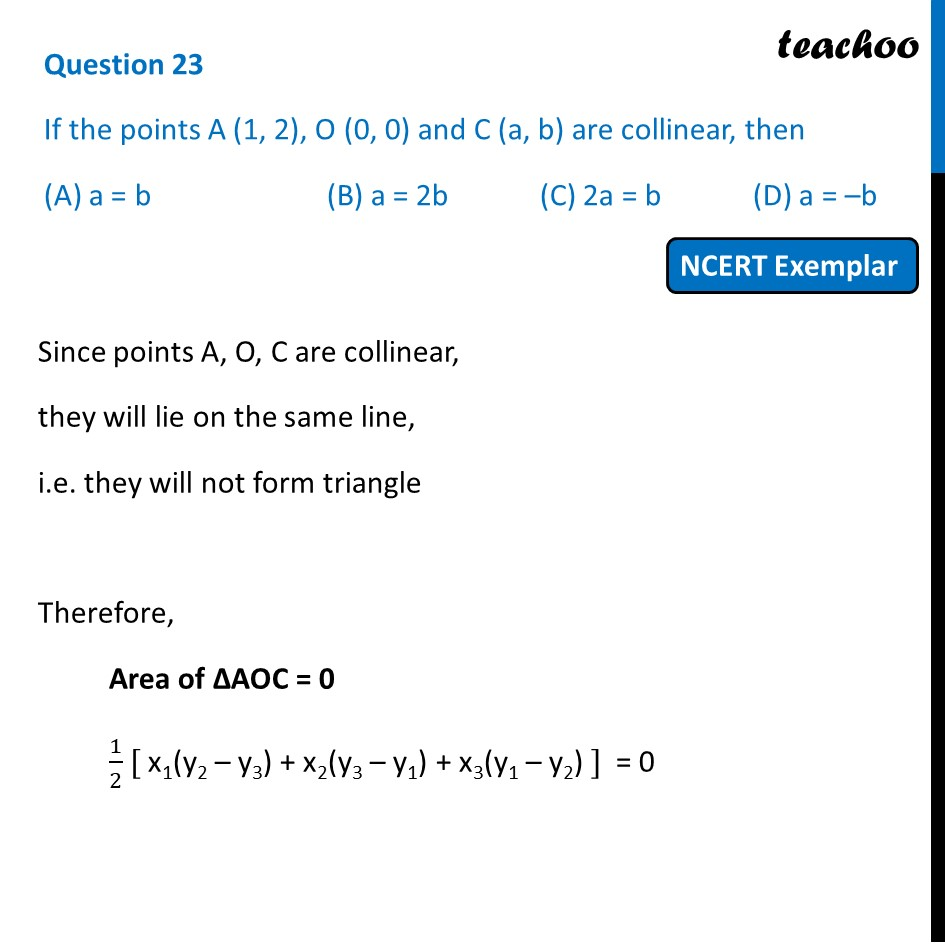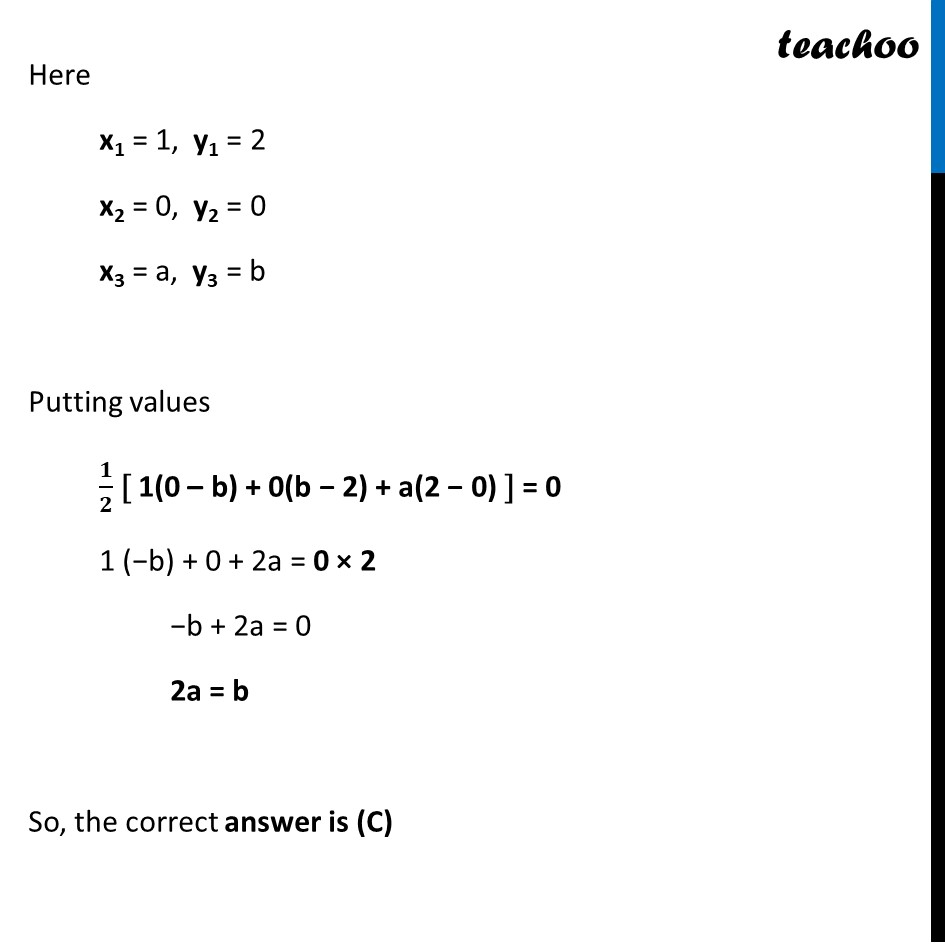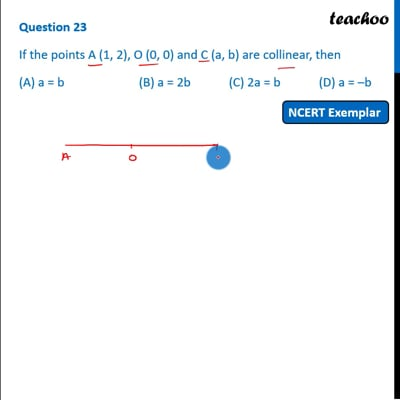NCERT Exemplar - MCQ

Chapter 7 Class 10 Coordinate Geometry
Serial order wise

## (D) a = –bThis video is only available for Teachoo black users

Introducing your new favourite teacher - Teachoo Black, at only ₹83 per month

### Transcript

Question 23 If the points A (1, 2), O (0, 0) and C (a, b) are collinear, then (A) a = b (B) a = 2b (C) 2a = b (D) a = –b Since points A, O, C are collinear, they will lie on the same line, i.e. they will not form triangle Therefore, Area of ∆AOC = 0 1/2 [ x1(y2 – y3) + x2(y3 – y1) + x3(y1 – y2) ] = 0 Here x1 = 1, y1 = 2 x2 = 0, y2 = 0 x3 = a, y3 = b Putting values 𝟏/𝟐 [ 1(0 – b) + 0(b − 2) + a(2 − 0) ] = 0 1 (−b) + 0 + 2a = 0 × 2 −b + 2a = 0 2a = b So, the correct answer is (C)